# Right asymptote of a simple function doesn't exist?

• lavoisier
In summary, the conversation discussed a problem related to oral absorption of drugs and the calculation of the right asymptote of a function A(t). It was found that the derivative of A(t) tends to -k for t→∞, but the intercept of the right asymptote was found to be infinite. The group discussed various examples and possible solutions to this issue. Ultimately, it was concluded that the existence of an oblique asymptote does not necessarily depend on the derivative of the function and may require alternative methods for determination.

#### lavoisier

Hi everyone, I was working on a problem recently, something related to oral absorption of drugs.
Cutting a long story short, at some point I needed to calculate the right asymptote of this function:

A(t) = Ln(k⋅t) - k⋅t

where k,t ∈ℝ+.

The derivative of A(t) tends to -k for t→∞, so I thought the right asymptote would be a line:

B(t)= m⋅t +q

with slope m = -k.

I went on to calculate the intercept q by the usual method, i.e. limit of A(t) + k⋅t for t→∞, and it turned out that it didn't exist (it said it was infinite)!

Is there any mistake in my calculations?
How can a curve have a finite, constant derivative for t→∞, suggesting that it approaches a line, but no intercept?

Tx
L

Yes, what you have is correct, The curve has asymptote y= -kx as x goes to infinity. But that is only for large t. The curve does not cross the x-axis because the function value is never positive. If f(t)= ln(kt)- kt, then f'(t)= (1/t)- k which is 0 at t= 1/k. At t= 1/k, kt= 1 so the function value is ln(1)- 1= 0- 1= -1. For 0< t< 1/k, k< 1/t so the derivative is positive. If 1/k< t the k> 1/t so the derivative is negative.

That is, as t increases from 0, the function values rises from negative infinity, to a maximum of -1, then decreases to negative infinity again getting closer and closer to the line y= -kx.

I think I didn't explain clearly enough what I meant, I wrote the post in a hurry.
It's not the intercept of A(t) that I was looking for, but the intercept of the right asymptote of A(t).
It doesn't look like the asymptote is simply -k t (i.e. a line with intercept = 0), as you can see from the plot (I chose k=1):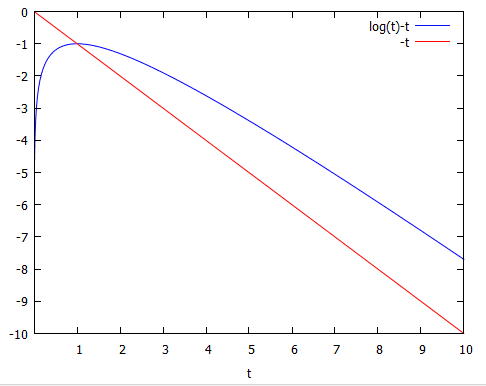In a related case, A(t) = Ln[exp(-k1 t)-exp(-k2 t)], with k2>k1, the asymptote is -k1 t, and that indeed matches what you see on the plot (k1=1, k2=2):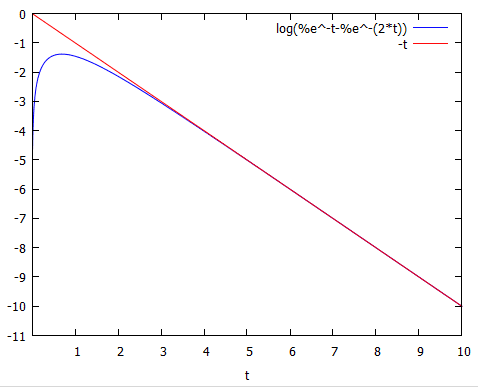So how can the asymptote of Ln(t)-t not have an intercept?
Because the limit of Ln(t) for t→∞ is ∞, but that doesn't make sense to me.
If this asymptote is a line with a finite slope -k (i.e. the line is not parallel to the y axis), it must cross the y-axis somewhere - or am I wrong?

lavoisier said:
A(t) = Ln(k⋅t) - k⋅t

where k,t ∈ℝ+.

The derivative of A(t) tends to -k for t→∞, so I thought the right asymptote would be a line:
There is something very wrong here.
• For t = k, the logarithm does not exist and for t>k it is not real.
• Let u = k - t. Then A(u)= ln(u)-u so dA/dt = dA/du*du/dt = (1/u-1)*(-1)=1 - 1/(k-t)
• Since A(t) does not exist for t≥k, letting t tend to ∞ has no meaning. The differential exists, though, and tends to 1.

Svein, I think you are misreading the function ##A(t)##. It is not ##\ln(k-t)##, but ##\ln(k\cdot t)##. As for lavoisier, I'm not quite sure how to solve your problem, but I did a quick check by substituting ##u=kt-1## so that I could write ##A(t)## as ##A(u)## in a form that works with the standard Taylor expansion of a logarithm. If you do this, it doesn't behave well (which isn't surprising since the series doesn't converge as ##t\rightarrow\infty##). I tried a few other things with hints from wikipedia, but I'm still getting poorly behaved series which is strange (I think) when a function's derivative has a limit at infinity.

DrewD said:
Svein, I think you are misreading the function A(t)A(t). It is not ln(kt)\ln(k-t), but ln(kt)\ln(k\cdot t).
Ah. That makes more sense. Then I agree that the derivative tends to -k. The second derivative is $\frac{-1}{t^{2}}$, which means that the curvature is downwards for all t. So - let the equation for the asymptote be $y= C - kt$ .
Since the curvature is downwards for all t, there should be no intersection between the original curve and the tangent.

Assuming an intersection, it would be at $y_{0}=C-kt_{0}=ln(kt_{0})-kt_{0}$. This gives $C=ln(kt_{0}) \therefore t_{0}=\frac{e^{C}}{k}$.

Thus, all lines with slope $- k$ intersects the original curve and cannot therefore be a tangent.

There are functions such that $lim_{x \rightarrow \infty} f(x) = m$ but $f(x)$ has no "oblique asymptote" as $x \rightarrow \infty$. The limit of the intercept $lim_{x\rightarrow \infty} (f(x) - mx)$ needs to exist in order to have an oblique asymptote.

The same is true for horizontal asymptotes. For example, $f(x) = ln(x)$ has no horizontal asymptote. If you imagine the graph of $ln(x)$ tilted by rotating it a little then you get an idea of what can happen in the oblique case.

If there is a goal to be accomplished by finding the oblique asymptote, perhaps it can be accomplished by other means.

OK, thank you all for the explanation.
I confess I can't visualise such a curve.
I really thought the intercept (or intersection with the y axis) *had* to exist unless the asymptote is a vertical line (i.e. a line with infinite slope).
For instance, I understand why y=x2 has no oblique asymptote: its derivative tends to ∞ for x→∞. Same for ex, Ln(x), etc...
But how a curve y=f(x) can have a finite derivative for x→∞, without having a right oblique asymptote, is something that escapes me.
Clearly I lack mathematical imagination. :O)

The derivative of ##\ln(x)## does not tend to ##\infty## as ##x\rightarrow \infty##. That is a simple example. This didn't occur to me earlier, but it should make it clear that there need not be an asymptote just because there is a finite derivative in the limit as ##x\rightarrow\infty##

That's true! Sorry, I hadn't though it through.
Still puzzled by the concept, but OK, that's just me.

The original problem I was trying to solve had nothing to do with asymptotes.
It's a problem one may encounter in predicting the efficacy of an orally administered drug (I am a medicinal chemist).
If you administer an oral dose D0 of a drug, it can be shown that under certain hypotheses its concentration in plasma C(t) is given by this function:

$C(t)=\frac{D_0 F} {V} \frac{k_a} {k_a-k}(e^{-k t}-e^{-k_a t})$

where F, k, ka and V are constants related to the absorption, elimination and distribution of the drug in the subject.

Here's the plot of the function for a typical case (D0=1 mg/kg, F=0.8, k=0.5 h-1, ka=1 h-1, V=2 L/kg). The time t is expressed in hours, and C(t) is expressed in mg/L: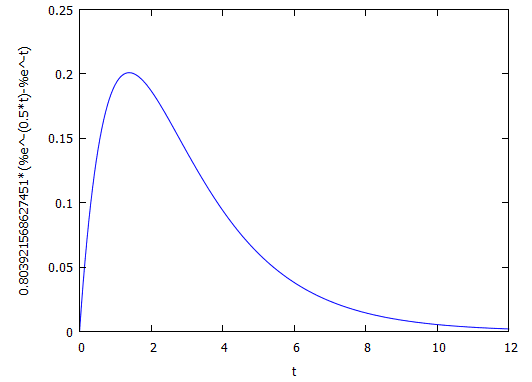To get the desired pharmacological effect, you often want this concentration to be above a given value (let's call it 'EC' or effective concentration) for a sufficiently long time. There are other metrics of efficacy, but this one ('time over EC') is very common.

So, say that in this case EC is 0.1 mg/L. If you add the EC line to the above graph: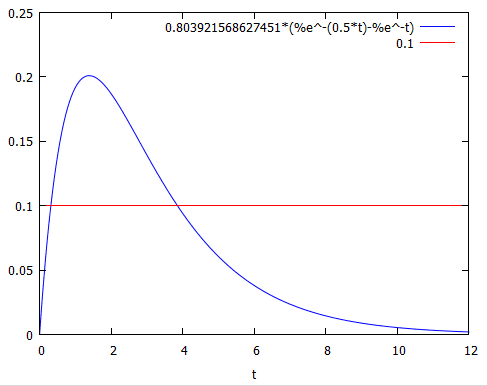you can see that the time over EC is a bit less than 4 h.
Now, this is fine if you have an actual curve and one value of EC. You can use a graphical method to find the intersections between the EC line and the curve, etc.

However, what if you want to study theoretically (and quantitatively) what happens to the 'time over EC' when you change the parameters (k, ka, V...).
As far as I know, to do that you should find an expression for 'time over EC' as a function of the parameters and EC, differentiate it w.r.t. each parameter and study the derivative.
'Time over EC' is clearly the difference between the two times where C(t) crosses the EC line.
That's where I got stuck. I don't think C(t)=EC can be solved analytically for t in general.

So my next step was to make an Excel file where you could see what happened to the curve when changing the various parameters.
That would be enough for most chemists, but I wanted to find an expression, at least an approximate one, for this flaming 'time over EC'.

So I tried to find an approximation to the C(t) curve that I could intersect analytically with EC.
The approach worked. I used the Pade approximation of the Taylor series centred on t=0 for the 'left' hand side of C(t) (before the max).
For the right hand side, I couldn't find a nice Taylor approximation, so I considered that Ln(C(t)) has a right asymptote that is reached quite early, and decided to intersect Ln(EC) with this asymptote. Here's the plot: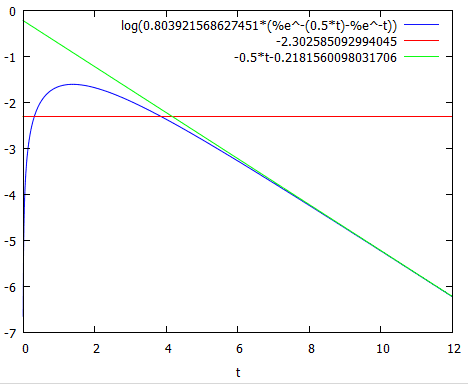Obviously there is a small error in using the asymptote rather than the actual curve, but nothing I couldn't live with.

And here we come to the problem I presented here.
When ka=k, C(t) can't be calculated with the usual function, it gives 0/0. If you take the limit of C(t) for ka→k, you find:

$C(t)=\frac{D_0 F} {V} k t e^{-k t}$

which is fine with Taylor on the left hand side, but on the right hand side gives the mess we just discussed.

What I find utterly crazy is that if I 'cheat' and use the original C(t) with ka≈k (e.g. 1.01 and 1, respectively), I get a curve that is almost identical to the one obtained with the new equation in k only, even in log scale: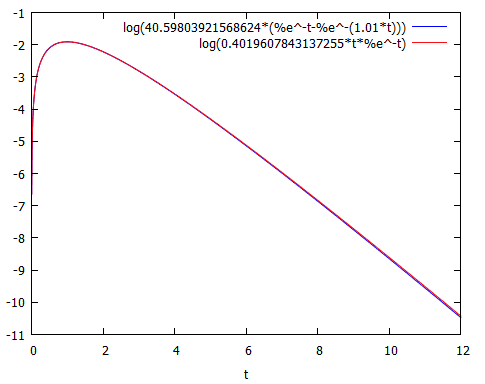Not unexpected, OK; but then I'm even more gobsmacked that the new equation should not allow asymptotes...

There you go, live and learn.
In practice, to approximate the 'time over EC' I think I'll use the curve where the constants are different, and in the cases where they are the same I will use two very close values for them. Quite distasteful, but what can you do...

Thanks again for your input, by the way.